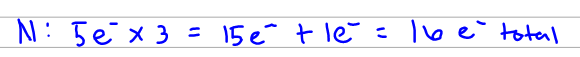# Problem: For the compound N3-:a. Draw the best Lewis structure.b. Determine the formal charge for all atoms.c. What is the electron domain geometry?d. What is the molecular shape?e. Is the molecule polar?f. What is the hybridization around the central atom?g. How many sigma bonds are present?h. How many pi bonds are present?

###### FREE Expert Solution
98% (132 ratings)
###### FREE Expert Solution

a. Draw the best Lewis structure.

We start by counting the valence electrons. We know that nitrogen has 5 e- each so we just multiply that by 3. Also, remember that we have a negative charge so we add one more efor that.98% (132 ratings)###### Problem Details

For the compound N3-:

a. Draw the best Lewis structure.

b. Determine the formal charge for all atoms.

c. What is the electron domain geometry?

d. What is the molecular shape?

e. Is the molecule polar?

f. What is the hybridization around the central atom?

g. How many sigma bonds are present?

h. How many pi bonds are present?

What scientific concept do you need to know in order to solve this problem?

Our tutors have indicated that to solve this problem you will need to apply the Hybridization concept. You can view video lessons to learn Hybridization. Or if you need more Hybridization practice, you can also practice Hybridization practice problems.

What is the difficulty of this problem?

Our tutors rated the difficulty ofFor the compound N3-:a. Draw the best Lewis structure.b. Det...as medium difficulty.

How long does this problem take to solve?

Our expert Chemistry tutor, Sabrina took 6 minutes and 45 seconds to solve this problem. You can follow their steps in the video explanation above.

What professor is this problem relevant for?

Based on our data, we think this problem is relevant for Professor Dixon's class at UCF.